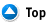Area
 Find the area of a: using the equation: Circle a =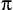r2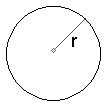Parallelogram a = bh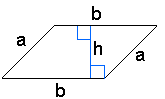Rectangle a = lw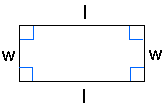Trapezoid a = 0.5h(b1 + b2)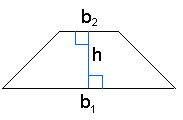Triangle a = 0.5bh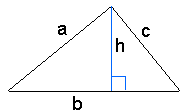Perimeter
 Find the perimeter of a: using the equation: Circle(The perimeter of a circle is called Circumference) c = 2r orc =*dParallelogram p = 2a + 2bRectangle p = 2l + 2wTrapezoidTriangle p = a + b + cVolume
 Find the volume of a: using the equation: Sphere v = 1.33**r3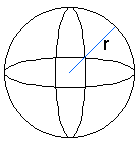Right Circular Cylinder v =r2h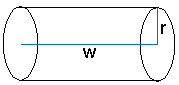Right Circular Cone v = .33**r2h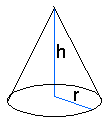Form of equation: Formula: Standard Form ax + by = c Slope Intercept Form/Y Intercept Form y = mx + b m = slopeb = y intercept X Intercept Form x = (y - b)/m b = y interceptm = slope Point-Slope Form y - y1 = m(x - x1) (x1,y1)m = slope